Courses

# Test: Power Semiconductor Diode & Transistors

## 10 Questions MCQ Test Topicwise Question Bank for Electronics Engineering | Test: Power Semiconductor Diode & Transistors

Description
This mock test of Test: Power Semiconductor Diode & Transistors for Electrical Engineering (EE) helps you for every Electrical Engineering (EE) entrance exam. This contains 10 Multiple Choice Questions for Electrical Engineering (EE) Test: Power Semiconductor Diode & Transistors (mcq) to study with solutions a complete question bank. The solved questions answers in this Test: Power Semiconductor Diode & Transistors quiz give you a good mix of easy questions and tough questions. Electrical Engineering (EE) students definitely take this Test: Power Semiconductor Diode & Transistors exercise for a better result in the exam. You can find other Test: Power Semiconductor Diode & Transistors extra questions, long questions & short questions for Electrical Engineering (EE) on EduRev as well by searching above.
QUESTION: 1

### In a Schottky diode junction is formed between

Solution:

In a Schottky diode junction is formed between metal and semiconductor

QUESTION: 2

### Assertion (A) : A Schottky diode has very high reverse voltage rating as compared to alloyed and diffused diodes. Reason (R) : In a Schottky diode the metallic layer is surrounded by a ring of insulating material such as SiO2.

Solution:

A Schottky diode has very low reverse voltage rating as compared to alloyed and diffused diodes. Maximum voltage rating is about 100 V. Thus, assertion is a false statement.
In a schottky diode, a thin epitaxial layer is grown on n-type base (substrate). On the epitaxial layer metal (gold, chromium or platinum) is deposited to form a thin layer. This metallic layer is surrounded by a ring of insulating material such as SiO2. Thus reason is a true statement.

QUESTION: 3

### Consider the following statements: 1. By increasing the rate of recombination and removal of minority carrier, reverse recovery period can be reduced. 2. In fast recovery diode, rate of recombination is decreased. 3. MOSFET and transistors are used in high power applications. 4. IGBT is having all the good properties of a transistors and MOSFET. Which of the statements given above are correct?

Solution:
• In fast recovery diode, reverse recovery time is reduced by addition of gold or platinum due to which rate of recombination is increased. Hence, statements-2 is not correct.
• MOSFET and transistor are used in low and medium power applications. Thus, statements-3 is not correct.
QUESTION: 4

A power transistor
1. cannot be easily paralleled.
2. is susceptible to secondary breakdown.
3. has low transconductance than MOSFET.
4. has negative temperature coefficient on collector current.
5. has low switching speed than MOSFET

Which of the above characteristics are possessed by a power transistor?

Solution:

A power transistor
1. can not be easily paralleled because of Vbe matching problems and local current concentration.
2. is susceptible to secondary breakdown.
3. has high transconductance.
4. has positive temperature coefficient on collector current since Ic in creases with temperature.
5. has low switching speed than MOSFET.

QUESTION: 5

Match List-I (Power Semiconductor Devices) with List-ll (Characteristics) and select the correct answer using the codes given below the lists:
List-I
A. Power BJT
B. Power MOSFET
C. IGBT
D. Schottky diode

List-ll
1. Exponential l-V characteristics
2. Suitable for high frequency inverters and choppers
3. Square law l-V characteristics
4. Offers ease of control and high current density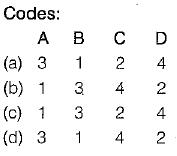Solution:
QUESTION: 6

Which of the following are not a unidirectional current devices?
1. BJT
2. Triac
3. MOSFET
4. GTO
5. IGBT

Solution:

Triac is a bidirectional device whereas BJT, MOSFET, GTO and IGBT are unidirectional devices.

QUESTION: 7

Match List-I (Power devices) with List-Il (Symbols) and select the correct answer using the codes given below the lists:
List-I
A. IGBT
B. MCT
C. Programmable Unijunction Transistor (PUT)
D. Silicon Controlled Switch (SCS)

List-II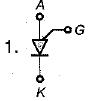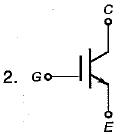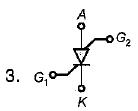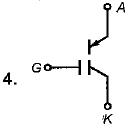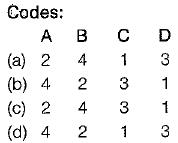Solution:
QUESTION: 8

Assertion (A): With increased reverse bias, the reverse blocking safe operating area increases in size.
Reason (R): The reverse blocking safe operating area is a plot of collector current versus collector-emitter voltage.

Solution:

The reverse blocking safe operating area (RBSOA) for a power transistor is shown below.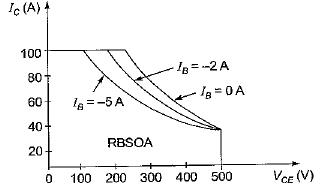It is clear from above curve that with increased reverse bias, the RBSOA decreases in size.

QUESTION: 9

A power transistor has its switching, waveforms as shown in figure. If the average power loss in the transistor is limited to 300 W, the switching frequency at which the transistor can be operated is approximately equal to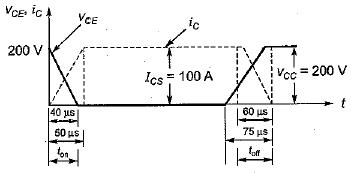Solution: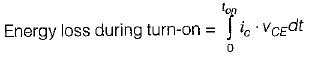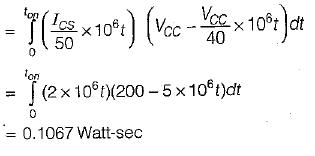Energy loss during turn-off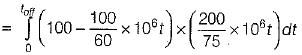= 0.1603 Watt-sec
Total energy loss in one cycle
= 0.1067 + 0.1603
= 0.267 W-sec
Average power loss in transistor
= Switching frequency x energy loss in one cycle
∴ Allowable switching frequency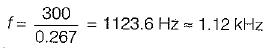QUESTION: 10

Match List-l (Power devices) with List-II (Applications) and select the correct answer using the codes given below the lists:
List-I
A. Schottky diode
B. BJT
C. IGBT
D. MOSFET

List-lI
1. SMPS
2. DC and AC motor drives
3. As a low input impedance device
4. High-frequency instrumentation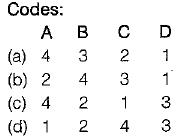Solution: